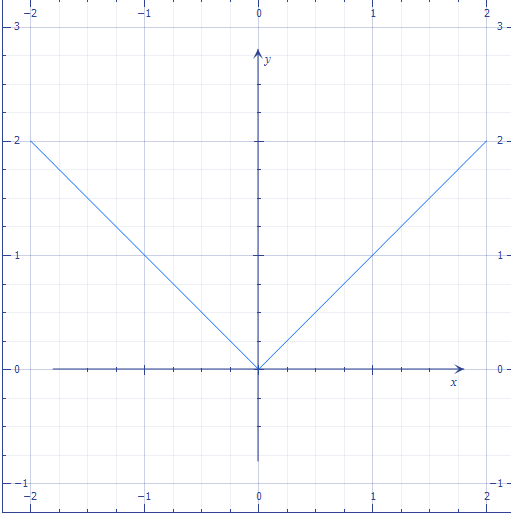# Tricky Corner!

Calculus Level 1Above shows the graph of $f(x) = | x|$, what is the value of $f'(0)$?

×